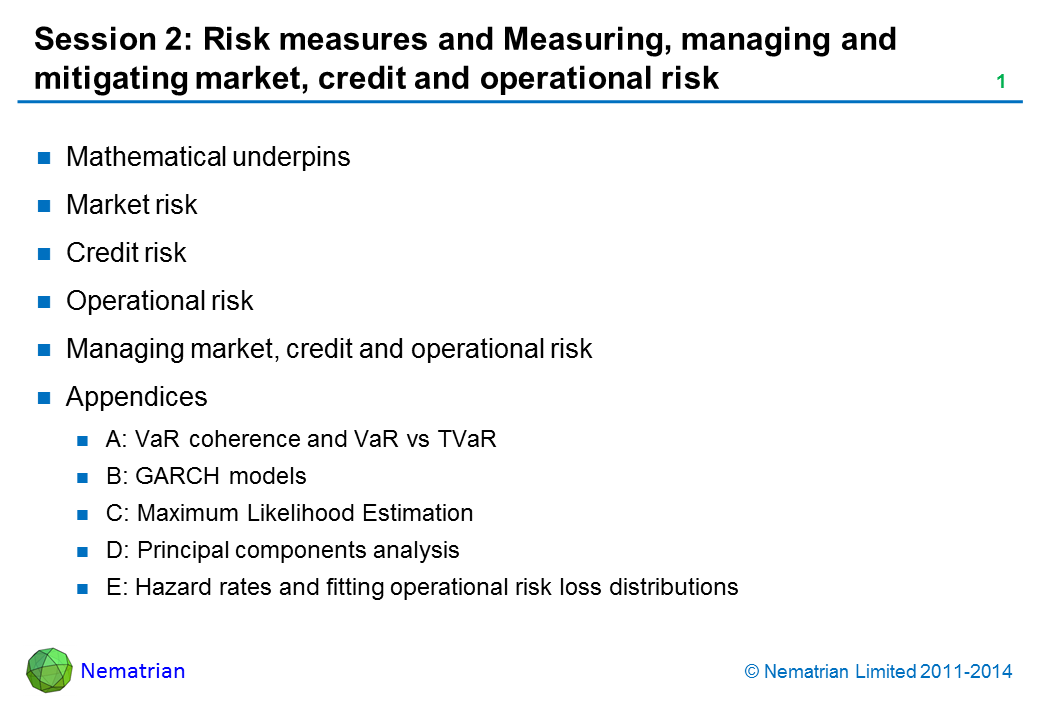/

### var x2=document.getElementById("RandomWalkHyperlink"); x2.setAttribute("width","250"); x2.setAttribute("height","30"); x2.setAttribute("style","border: 1px solid black"); var c2=x2.getContext("2d"); var sigma=.06; var mu=0; var y=new Array; var r=new Array; var ylength=100; var i; var xplotlow=2; var xplothigh=80; var yplotlow=x2.height-2; var yplothigh=6; var frameRate=100; var lineColour="red" y=1; for (i=1;i<ylength;i++) { r[i]=inverseNormal(Math.random()); y[i]=y[i-1]*Math.exp(mu+sigma*r[i]); } projectLineChartOntoCanvas(c2,y,xplotlow,xplothigh,yplotlow,yplothigh,lineColour); animateRandomWalk(x2,c2,y,xplotlow,xplothigh,yplotlow,yplothigh,lineColour,mu,sigma,frameRate) function animateRandomWalk(canvas,ctx,y,xplotlow,xplothigh,yplotlow,yplothigh,strokeStyle,mu,sigma,frameRate) { var i; var plotID=null; plotIncrement(); plotID=setInterval(plotIncrement,frameRate); function plotIncrement() { ctx.fillStyle="white"; ctx.fillRect(0,0,canvas.width,canvas.height); ctx.clearRect(0,0,canvas.width,canvas.height); var i; var ylength=y.length; for (i=0;i<ylength-1;i++) { y[i]=y[i+1]; } y[ylength-1]=y[ylength-2]*Math.exp(mu+sigma*inverseNormal(Math.random())); ctx.beginPath(); projectLineChartOntoCanvas(ctx,y,xplotlow,xplothigh,yplotlow,yplothigh,strokeStyle); c2.fillStyle="maroon"; c2.font="16px Arial"; c2.fillText("ERM Resources",110,21); } } function projectLineChartOntoCanvas(ctx,y,xplotlow,xplothigh,yplotlow,yplothigh,strokeStyle) { var i,ylow,yhigh,xplotscale,yplotscale; var xplot=new Array; var yplot=new Array; ylength=y.length; ylow=0; yhigh=0; for (i=0;i<ylength;i++) { if (y[i]<ylow) { ylow=y[i];} if (y[i] > yhigh) { yhigh=y[i];} } xplotscale=(xplothigh-xplotlow)/(ylength-1); yplotscale=(yplothigh-yplotlow)/(yhigh-ylow); for (i=0;i<ylength;i++) { xplot[i]=xplotlow+i*xplotscale; yplot[i]=yplotlow+y[i]*yplotscale; } ctx.beginPath(); ctx.strokeStyle=strokeStyle; ctx.moveTo(xplot,yplot); for (i=1;i<ylength;i++) { ctx.lineTo(xplot[i],yplot[i]); } ctx.stroke(); ctx.beginPath(); ctx.strokeStyle="green"; ctx.lineWidth=1; ctx.strokeRect(xplotlow,Math.min(yplotlow,yplothigh)-4,xplothigh-xplotlow,Math.abs(yplothigh-yplotlow)+4); ctx.stroke(); } function inverseNormal(x){ // Adapted from Peter Acklam's "An algorithm for computing the inverse normal cumulative distribution function" var a1=-39.6968302866538; var a2=220.946098424521; var a3=-275.928510446969; var a4=138.357751867269; var a5=-30.6647980661472; var a6=2.50662827745924; var b1=-54.4760987982241; var b2=161.585836858041; var b3=-155.698979859887; var b4=66.8013118877197; var b5=-13.2806815528857; var c1=-0.00778489400243029; var c2=-0.322396458041136; var c3=-2.40075827716184; var c4=-2.54973253934373; var c5=4.37466414146497; var c6=2.93816398269878; var d1=0.00778469570904146; var d2=0.32246712907004; var d3=2.445134137143; var d4=3.75440866190742; var x_low=0.02425; var x_high=1-x_low; var q,r,ans; if (x <= 0) { throw "InverseNormal: argument outside valid range" } else if (x<x_low) { q=Math.sqrt(-2*Math.log(x)) return (((((c1*q+c2)*q+c3)*q+c4)*q+c5)*q+c6)/ ((((d1*q+d2)*q+d3)*q+d4)*q+1) } else if (x <= x_high) { q=x-0.5 r=q*q return (((((a1*r+a2)*r+a3)*r+a4)*r+a5)*r+a6)*q/ (((((b1*r+b2)*r+b3)*r+b4)*r+b5)*r+1) } else if (x<1) { q=Math.sqrt(-2*Math.log(1-x)) return -(((((c1*q+c2)*q+c3)*q+c4)*q+c5)*q+c6)/ ((((d1*q+d2)*q+d3)*q+d4)*q+1) } else { throw "InverseNormal: argument outside valid range" } } Measuring and managing market, credit and Op risk 

Go to: Summary | Next